JMSLTM Numerical Library 7.2.0
com.imsl.datamining.decisionTree

## Class DecisionTree

• All Implemented Interfaces:
Serializable, Cloneable
Direct Known Subclasses:
CHAID, DecisionTreeInfoGain, QUEST

```public abstract class DecisionTree
extends PredictiveModel
implements Serializable, Cloneable```

Abstract class for generating a decision tree for a single response variable and one or more predictor variables.

### Tree Generation Methods

This package contains four of the most widely used algorithms for decision trees (`C45`, `ALACART`, `CHAID`, and `QUEST`). The user may also provide an alternate algorithm by extending the `DecisionTree` or `DecisionTreeInfoGain` abstract class and implementing the abstract method ```selectSplitVariable( double[][], double[], double[], double[], int[])```.

### Optimal Tree Size

#### Minimum Cost-complexity Pruning

A strategy to address overfitting is to grow the tree as large as possible, and then use some logic to prune it back. Let T represent a decision tree generated by any of the methods above. The idea (from Breiman, et. al.) is to find the smallest sub-tree of T that minimizes the cost-complexity measure: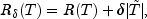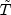denotes the set of terminal nodes.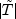represents the number of terminal nodes, and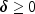is a cost-complexity parameter. For a categorical target variable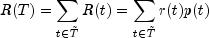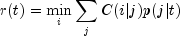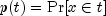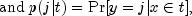and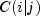is the cost for misclassifying the actual class j as i. Note that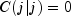and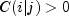, for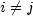.

When the target is continuous (and the problem is a regression problem), the metric is instead the mean squared error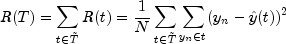This class implements the optimal pruning algorithm 10.1, page 294 in Breiman, et. al (1984). The result of the algorithm is a sequence of sub-trees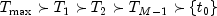obtained by pruning the fully generated tree,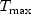, until the sub-tree consists of the single root node,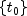. Corresponding to the sequence of sub-trees is the sequence of complexity values,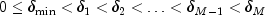where M is the number of steps it takes in the algorithm to reach the root node. The sub-trees represent the optimally-pruned sub-trees for the sequence of complexity values. The minimum complexity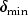can be set via an optional argument.

#### V-Fold Cross-Validation

The `CrossValidation` class can be used for model validation.

### Prediction

#### Bagging

The `BootstrapAggregation` class provides predictions through a resampling scheme.

#### Missing Values

Any observation or case with a missing response variable is eliminated from the analysis. If a predictor has a missing value, each algorithm will skip that case when evaluating the given predictor. When making a prediction for a new case, if the split variable is missing, the prediction function applies surrogate split-variables and splitting rules in turn, if they are estimated with the decision tree. Otherwise, the prediction function returns the prediction from the most recent non-terminal node. In this implementation, only `ALACART` estimates surrogate split variables when requested.

Example 1, Example 2, Example 3, Example 4, Serialized Form
• ### Nested Class Summary

Nested Classes
Modifier and Type Class and Description
`static class ` `DecisionTree.MaxTreeSizeExceededException`
Exception thrown when the maximum tree size has been exceeded.
`static class ` `DecisionTree.PruningFailedToConvergeException`
Exception thrown when pruning fails to converge.
`static class ` `DecisionTree.PureNodeException`
Exception thrown when attempting to split a node that is already pure (response variable is constant).
• ### Nested classes/interfaces inherited from class com.imsl.datamining.PredictiveModel

`PredictiveModel.PredictiveModelException, PredictiveModel.StateChangeException, PredictiveModel.SumOfProbabilitiesNotOneException, PredictiveModel.VariableType`
• ### Constructor Summary

Constructors
Constructor and Description
```DecisionTree(double[][] xy, int responseColumnIndex, PredictiveModel.VariableType[] varType)```
Constructs a `DecisionTree` object for a single response variable and multiple predictor variables.
• ### Method Summary

Methods
Modifier and Type Method and Description
`void` `fitModel()`
Fits the decision tree.
`double[]` `getCostComplexityValues()`
Returns an array containing cost-complexity values.
`Tree` `getDecisionTree()`
Returns a `Tree` object.
`double` `getFittedMeanSquaredError()`
Returns the mean squared error on the training data.
`int` `getMaxDepth()`
Returns the maximum depth a tree is allowed to have.
`int` `getMaxNodes()`
Returns the maximum number of `TreeNode` instances allowed in a tree.
`double` `getMeanSquaredPredictionError()`
Returns the mean squared error.
`int` `getMinObsPerChildNode()`
Returns the minimum number of observations that are required for any child node before performing a split.
`int` `getMinObsPerNode()`
Returns the minimum number of observations that are required in a node before performing a split.
`int[]` `getNodeAssigments(double[][] testData)`
Returns the terminal node assignments for each row of the test data.
`int` `getNumberOfComplexityValues()`
Return the number of cost complexity values determined.
`protected int` ```getNumberOfSets(double[] parentFreqs, int[] splita)```
Returns the number of sets for a split.
`boolean` `isAutoPruningFlag()`
Returns the auto-pruning flag.
`double[]` `predict()`
Predicts the training examples (in-sample predictions) using the most recently grown tree.
`double[]` `predict(double[][] testData)`
Predicts new data using the most recently grown decision tree.
`double[]` ```predict(double[][] testData, double[] testDataWeights)```
Predicts new weighted data using the most recently grown decision tree.
`void` `printDecisionTree(boolean printMaxTree)`
Prints the contents of the Decision Tree using distinct but general labels.
`void` ```printDecisionTree(String responseName, String[] predictorNames, String[] classNames, String[] categoryNames, boolean printMaxTree)```
Prints the contents of the Decision Tree.
`void` `pruneTree(double gamma)`
Finds the minimum cost-complexity decision tree for the cost-complexity value, gamma.
`protected abstract int` ```selectSplitVariable(double[][] xy, double[] classCounts, double[] parentFreq, double[] splitValue, int[] splitPartition)```
Abstract method for selecting the next split variable and split definition for the node.
`void` `setAutoPruningFlag(boolean autoPruningFlag)`
Sets the flag to automatically prune the tree during the fitting procedure.
`protected void` `setConfiguration(PredictiveModel pm)`
Sets the configuration of `PredictiveModel` to that of the input model.
`void` `setCostComplexityValues(double[] gammas)`
Sets the cost-complexity values.
`void` `setMaxDepth(int nLevels)`
Specifies the maximum tree depth allowed.
`void` `setMaxNodes(int maxNodes)`
Sets the maximum number of nodes allowed in a tree.
`void` `setMinCostComplexityValue(double minCostComplexity)`
Sets the value of the minimum cost-complexity value.
`void` `setMinObsPerChildNode(int nObs)`
Specifies the minimum number of observations that a child node must have in order to split, one of several tree size and splitting control parameters.
`void` `setMinObsPerNode(int nObs)`
Specifies the minimum number of observations a node must have to allow a split, one of several tree size and splitting control parameters.
• ### Methods inherited from class com.imsl.datamining.PredictiveModel

`getClassCounts, getCostMatrix, getMaxNumberOfCategories, getNumberOfClasses, getNumberOfColumns, getNumberOfMissing, getNumberOfPredictors, getNumberOfRows, getNumberOfUniquePredictorValues, getPredictorIndexes, getPredictorTypes, getPrintLevel, getPriorProbabilities, getRandomObject, getResponseColumnIndex, getResponseVariableAverage, getResponseVariableMostFrequentClass, getResponseVariableType, getTotalWeight, getVariableType, getWeights, getXY, isMustFitModelFlag, isUserFixedNClasses, setClassCounts, setCostMatrix, setFitModelFlag, setMaxNumberOfCategories, setNumberOfClasses, setPredictorIndex, setPredictorTypes, setPrintLevel, setPriorProbabilities, setRandomObject, setWeights`
• ### Methods inherited from class java.lang.Object

`clone, equals, finalize, getClass, hashCode, notify, notifyAll, toString, wait, wait, wait`
• ### Constructor Detail

• #### DecisionTree

```public DecisionTree(double[][] xy,
int responseColumnIndex,
PredictiveModel.VariableType[] varType)```
Constructs a `DecisionTree` object for a single response variable and multiple predictor variables.
Parameters:
`xy` - a `double` matrix with rows containing the observations on the predictor variables and one response variable.
`responseColumnIndex` - an `int` specifying the column index of the response variable.
`varType` - a `PredictiveModel.VariableType` array containing the type of each variable.
• ### Method Detail

• #### fitModel

```public void fitModel()
throws PredictiveModel.PredictiveModelException,
DecisionTree.PruningFailedToConvergeException,
PredictiveModel.StateChangeException,
DecisionTree.PureNodeException,
PredictiveModel.SumOfProbabilitiesNotOneException,
DecisionTree.MaxTreeSizeExceededException```
Fits the decision tree. Implements the abstract method.
Overrides:
`fitModel` in class `PredictiveModel`
Throws:
`PredictiveModel.PredictiveModelException` - an exception has occurred in the com.imsl.datamining.PredictiveModel. Superclass exceptions should be considered such as com.imsl.datamining.PredictiveModel.StateChangeException and com.imsl.datamining.PredictiveModel.SumOfProbabilitiesNotOneException}.
`DecisionTree.PruningFailedToConvergeException` - pruning has failed to converge.
`PredictiveModel.StateChangeException` - an input parameter has changed that might affect the model estimates or predictions.
`DecisionTree.PureNodeException` - attempting to split a node that is already pure.
`PredictiveModel.SumOfProbabilitiesNotOneException` - the sum of probabilities is not approximately one.
`DecisionTree.MaxTreeSizeExceededException` - the maximum tree size has been exceeded.
• #### getCostComplexityValues

```public double[] getCostComplexityValues()
throws DecisionTree.PruningFailedToConvergeException,
PredictiveModel.StateChangeException```
Returns an array containing cost-complexity values.
Returns:
a `double` array containing the cost-complexity values.

The cost-complexity values are found via the optimal pruning algorithm of Breiman, et. al.

Throws:
`DecisionTree.PruningFailedToConvergeException` - pruning has failed to converge.
`PredictiveModel.StateChangeException` - an input parameter has changed that might affect the model estimates or predictions.
• #### getDecisionTree

```public Tree getDecisionTree()
throws PredictiveModel.StateChangeException```
Returns a `Tree` object.
Returns:
a `Tree` object containing the tree structure information.
Throws:
`PredictiveModel.StateChangeException` - an input parameter has changed that might affect the model estimates or predictions.
• #### getFittedMeanSquaredError

```public double getFittedMeanSquaredError()
throws PredictiveModel.StateChangeException```
Returns the mean squared error on the training data.
Returns:
a `double` equal to the mean squared error between the fitted value and the actual value of the response variable in the training data.
Throws:
`PredictiveModel.StateChangeException` - an input parameter has changed that might affect the model estimates or predictions.
• #### getMaxDepth

`public int getMaxDepth()`
Returns the maximum depth a tree is allowed to have.
Returns:
an `int` indicating the maximum depth a tree is allowed to have.
• #### getMaxNodes

`public int getMaxNodes()`
Returns the maximum number of `TreeNode` instances allowed in a tree.
Returns:
an `int` indicating the maximum number of nodes allowed in a tree.
• #### getMeanSquaredPredictionError

```public double getMeanSquaredPredictionError()
throws PredictiveModel.StateChangeException```
Returns the mean squared error.
Returns:
a `double` equal to the mean squared error between the predicted value and the actual value of the response variable. The error is the in-sample fitted error if `predict` is first called with no arguments. Otherwise, the error is relative to the test data provided in the call to `predict`.
Throws:
`PredictiveModel.StateChangeException` - an input parameter has changed that might affect the model estimates or predictions.
• #### getMinObsPerChildNode

`public int getMinObsPerChildNode()`
Returns the minimum number of observations that are required for any child node before performing a split.
Returns:
an `int` indicating the minimum number of observations that are required for any child node before performing a split.
• #### getMinObsPerNode

`public int getMinObsPerNode()`
Returns the minimum number of observations that are required in a node before performing a split.
Returns:
an `int` indicating the minimum number of observations that are required in a node before performing a split.
• #### getNodeAssigments

`public int[] getNodeAssigments(double[][] testData)`
Returns the terminal node assignments for each row of the test data.
Parameters:
`testData` - a `double` matrix containing the test data

`testData` must have the same column structure and type as the training data.

Returns:
an `int` array containing the (0-based) terminal node id's for each observation in `testData`
• #### getNumberOfComplexityValues

`public int getNumberOfComplexityValues()`
Return the number of cost complexity values determined.
Returns:
an `int` indicating the number of cost complexity values determined.
• #### getNumberOfSets

```protected int getNumberOfSets(double[] parentFreqs,
int[] splita)```
Returns the number of sets for a split.
Parameters:
`parentFreqs` - a `double` array containing frequencies of the response variable in the data subset of the parent node.
`splita` - an `int` array that contains the split partition determined in the `selectSplitVariable(double[][], double[], double[], double[], int[])` method.
Returns:
an `int` that is the number of sets.
• #### isAutoPruningFlag

`public boolean isAutoPruningFlag()`
Returns the auto-pruning flag. See description `setAutoPruningFlag(boolean)` for details.
Returns:
a `boolean` which if `true` means that the model is configured to perform automatic pruning.
• #### predict

```public double[] predict()
throws PredictiveModel.StateChangeException```
Predicts the training examples (in-sample predictions) using the most recently grown tree.
Specified by:
`predict` in class `PredictiveModel`
Returns:
a `double` array of fitted values of the response variable using the most recently grown decision tree. To populate fitted values, use the `predict` method without arguments.
Throws:
`PredictiveModel.StateChangeException` - an input parameter has changed that might affect the model estimates or predictions.
• #### predict

```public double[] predict(double[][] testData)
throws PredictiveModel.StateChangeException```
Predicts new data using the most recently grown decision tree.
Specified by:
`predict` in class `PredictiveModel`
Parameters:
`testData` - a `double` matrix containing test data for which predictions are to be made using the current tree. `testData` must have the same number of columns and the columns must be in the same arrangement as `xy`.
Returns:
a `double` array of predicted values of the response variable using the most recently grown decision tree.
Throws:
`PredictiveModel.StateChangeException` - an input parameter has changed that might affect the model estimates or predictions.
• #### predict

```public double[] predict(double[][] testData,
double[] testDataWeights)
throws PredictiveModel.StateChangeException```
Predicts new weighted data using the most recently grown decision tree.
Specified by:
`predict` in class `PredictiveModel`
Parameters:
`testData` - a `double` matrix containing test data for which predictions are to be made using the current tree. `testData` must have the same number of columns and the columns must be in the same arrangement as `xy`.
`testDataWeights` - a `double` array containing weights for each row of `testData`.
Returns:
a `double` array of predicted values of the response variable using the most recently grown decision tree.
Throws:
`PredictiveModel.StateChangeException` - an input parameter has changed that might affect the model estimates or predictions.
• #### printDecisionTree

`public void printDecisionTree(boolean printMaxTree)`
Prints the contents of the Decision Tree using distinct but general labels.

This method uses default values for the variable labels when printing (see `printDecisionTree` (String, String[], String[], String[], boolean) for these values.)

Parameters:
`printMaxTree` - a `boolean` indicating that the maximal tree should be printed.

Otherwise the pruned tree is printed.

• #### printDecisionTree

```public void printDecisionTree(String responseName,
String[] predictorNames,
String[] classNames,
String[] categoryNames,
boolean printMaxTree)```
Prints the contents of the Decision Tree.
Parameters:
`responseName` - a `String` specifying a name for the response variable.

If `null`, the default value is used.

Default: `responseName` = Y

`predictorNames` - a `String` array specifying names for the response variables.

If `null`, the default value is used.

Default: `predictorNames` = X0, X1, ...

`classNames` - a `String` array specifying names for the classes.

If `null`, the default value is used.

Default: `classNames` = 0, 1, 2, ...

`categoryNames` - a `String` array specifying names for the categories.

If `null`, the default value is used.

Default: `categoryNames` = 0, 1, 2, ...

`printMaxTree` - a `boolean` indicating that the maximal tree should be printed.

Otherwise the pruned tree is printed.

• #### pruneTree

`public void pruneTree(double gamma)`
Finds the minimum cost-complexity decision tree for the cost-complexity value, gamma.

The method implements the optimal pruning algorithm 10.1, page 294 in Breiman, et. al (1984). The result of the algorithm is a sequence of sub-treesobtained by pruning the fully generated tree,, until the sub-tree consists of the single root node,. Corresponding to the sequence of sub-trees is the sequence of complexity values,where M is the number of steps it takes in the algorithm to reach the root node. The sub-trees represent the optimally pruned sub-trees for the sequence of complexity values.

The effect of the pruning is stored in the tree's terminal node array. That is, when the algorithm determines that the tree should be pruned at a particular node, it sets that node to be a terminal node using the method `Tree.setTerminalNode(int, boolean)`. No other changes are made to the tree structure so that the maximal tree can still be printed and reviewed. However, once a tree is pruned, all the predictions will use the pruned tree.
Parameters:
`gamma` - a `double` equal to the cost-complexity parameter.
• #### selectSplitVariable

```protected abstract int selectSplitVariable(double[][] xy,
double[] classCounts,
double[] parentFreq,
double[] splitValue,
int[] splitPartition)```
Abstract method for selecting the next split variable and split definition for the node.
Parameters:
`xy` - a `double` matrix containing the data.
`classCounts` - a `double` array containing the counts for each class of the response variable, when it is categorical.
`parentFreq` - a `double` array used to indicate which subset of the observations belong in the current node.
`splitValue` - a `double` array representing the resulting split point if the selected variable is quantitative.
`splitPartition` - an `int` array indicating the resulting split partition if the selected variable is categorical.
Returns:
an `int` specifying the column index of the split variable in `xy`.
• #### setAutoPruningFlag

`public void setAutoPruningFlag(boolean autoPruningFlag)`
Sets the flag to automatically prune the tree during the fitting procedure.

The default value is `false`. Set to `true` before calling `fitModel()` in order to prune the tree automatically. The pruning will use the cost-complexity value equal to `minCostComplexityValue`. See also `pruneTree(double)` which prunes the tree using a given cost-complexity value.

Parameters:
`autoPruningFlag` - a `boolean` value that when `true` indicates that the maximally grown tree should be automatically pruned in `fitModel()`.

Default: `autoPruningFlag`=`false`.

• #### setConfiguration

```protected void setConfiguration(PredictiveModel pm)
throws DecisionTree.PruningFailedToConvergeException,
PredictiveModel.StateChangeException,
PredictiveModel.SumOfProbabilitiesNotOneException```
Sets the configuration of `PredictiveModel` to that of the input model.
Specified by:
`setConfiguration` in class `PredictiveModel`
Parameters:
`pm` - a `PredictiveModel` object which is to have its attributes duplicated in this instance.
Throws:
`DecisionTree.PruningFailedToConvergeException` - pruning has failed to converge.
`PredictiveModel.StateChangeException` - an input parameter has changed that might affect the model estimates or predictions.
`PredictiveModel.SumOfProbabilitiesNotOneException` - the sum of the probabilities does not equal 1.
• #### setCostComplexityValues

`public void setCostComplexityValues(double[] gammas)`
Sets the cost-complexity values. For the original tree, the values are generated in `fitModel()` when `isAutoPruningFlag()` returns `true`.
Parameters:
`gammas` - `double` array containing cost-complexity values. This method is used when copying the configuration of one tree to another.

Default: `gammas` = `setMinCostComplexityValue(double)`.

• #### setMaxDepth

`public void setMaxDepth(int nLevels)`
Specifies the maximum tree depth allowed.
Parameters:
`nLevels` - an `int` specifying the maximum depth that the `DecisionTree` is allowed to have. `nLevels` should be strictly positive.

Default: `nLevels` = 10.

• #### setMaxNodes

`public void setMaxNodes(int maxNodes)`
Sets the maximum number of nodes allowed in a tree.
Parameters:
`maxNodes` - an `int` specifying the maximum number of nodes allowed in a tree.

Default: `maxNodes` = 100.

• #### setMinCostComplexityValue

`public void setMinCostComplexityValue(double minCostComplexity)`
Sets the value of the minimum cost-complexity value.
Parameters:
`minCostComplexity` - a `double` indicating the smallest value to use in cost-complexity pruning. The value must be in [0.0, 1.0].

Default: `minCostComplexity` = 0.

• #### setMinObsPerChildNode

`public void setMinObsPerChildNode(int nObs)`
Specifies the minimum number of observations that a child node must have in order to split, one of several tree size and splitting control parameters.
Parameters:
`nObs` - an `int` specifying the minimum number of observations that a child node must have in order to split the current node. `nObs` must be strictly positive. `nObs` must also be greater than the minimum number of observations required before a node can split `setMinObsPerNode(int)`.

Default: `nObs` = 7.

• #### setMinObsPerNode

`public void setMinObsPerNode(int nObs)`
Specifies the minimum number of observations a node must have to allow a split, one of several tree size and splitting control parameters.
Parameters:
`nObs` - an `int` specifying the number of observations the current node must have before considering a split. `nObs` should be greater than 1 but less than or equal to the number of observations in `xy`.

Default: `nObs` = 21.

JMSLTM Numerical Library 7.2.0